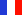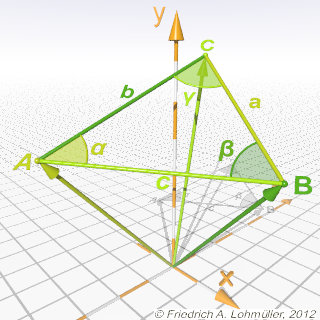###### Descriptions and Examples for the POV-Ray Raytracerby Friedrich A. Lohmüller Elementary Geometry for Raytracing
ItalianoFrançaisDeutschHome
- POV-Ray Tutorial

- Geometrical Basics
for Raytracing

Right-angled Triangle
Pythagorean Theorem
Trigonometry Basics
Law of cosines
Equilateral Triangle
Regular Polygon
Polyhedron
Tetrahedron
Octahedron
Cube & Cuboid
Dodecahedron
Icosahedron
Cuboctahedron
Truncated Octahedron
Rhombicuboctahedron
Truncated Icosahedron
Circles
Tangent circles
Internal Tangents
External Tangents

- Geometric 3D Animations

## The Law of CosinesSome useful geometrical facts on the sides and angles of triangles.

Note: The trigonometric functions sin(X), cos(X) and tan(X) in POV-Ray
need their arguments X in radians !!!   The symbole π = pi in POV-Ray.
According to this the reverse fuctions asin(x), acos(x) and atan(x) are giving the angles in radians.

 For every tiangle ABC we have:   c2 = a2 + b2 - 2*a*b*cos( γ )   (1)   b2 = a2 + c2 - 2*a*c*cos( β )   (2)   a2 = b2 + c2 - 2*b*c*cos( α )   (3) For γ = 90° = pi/2 (right-angled triangle) we have cos(γ) = 0 and for this by (1): c2 = a2 + b2 (Pythagorean Theorem). ----------------------------------------------------- For the angles of a triangle ABC we get the following formulas: γ = acos ( ( a2 + b2 - c2 )/(2*a*b) )   (4) β = acos ( ( a2 + c2 - b2 )/ (2*a*c) )   (5) α = acos ( ( b2 + c2 - a2 )/ (2*b*c) )   (6)A triangle ABC in 2D.A triangle ABC in 3D.
top

 © Friedrich A. Lohmüller, 2014 www.f-lohmueller.de